A COMPUTATIONAL SOFTWARE FOR NOISE MEASUREMENT AND TOWARD ITS IDENTIFICATION

M. SAKURAI
Yoshimasa Electronic Inc., Daiichi-Nishiwaki Bldg., 1-58-10 Yoyogi, Shibuya, Tokyo, 151-0053 Japan
E-mail: saku@ymec.com

AND

H. SAKAI AND Y. ANDO
Graduate School of Science and Technology, Kobe University, Rokkodai, Nada, Kobe, 657-8501 Japan

The computational system outlined here is based on a model of the human auditory-brain system including the autocorrelation and interaural crosscorrelation mechanisms, and the specialization of the cerebral hemispheres . It consists of a binaural receiver, a laptop computer, and software designed to measure the physical factors of noise fields and to identify environmental noise. The temporal factors te, t1 and f1 as well as F(0), are extracted from the autocorrelation function (ACF) of the noise source, and the spatial factors LL, IACC, tIACC, and WIACC are extracted from the interaural crosscorrelation function (IACF). These factors may be utilized for subjective evaluations of a source of noise and a noise field.

1. INTRODUCTION

For many years, environmental noise has been evaluated in terms of the statistical sound pressure level (SPL), represented as Lx or Leq, and its power spectrum measured by a monaural sound level meter. The SPL and power spectrum alone, however, do not provide a description that matches subjective evaluations of environmental noise. Descriptions of many subjective attributes such as preference and diffuseness, as well as primary sensations (loudness, pitch, and timbre), can be based on a model of the response of the human auditory-brain system to sound fields , and the predictions of that model have been found to be consistent with experimental results. The loudness of band-limited noise, for example, has recently been shown to be affected by the effective duration of the autocorrelation function (ACF), te, as well as by the SPL [2, 3]. When a fundamental frequency of complex tones is below about 1200 Hz, the pitch and its strength are indicated well by t1 and f1 respectively . In particular, the ACF factors obtained at (te)min are good indicators of differences in the subjective evaluation of the noise source and the noise field [5, 6].
The model consists of autocorrelators on the signals at two auditory pathways and an interaural crosscorrelator between then signals, and it takes into account the specialization of the cerebral hemispheres in humans. The ACF and interaural crosscorrelation function (IACF) of sound signals arriving at both ears are calculated. Orthogonal factors F(0), te, t1, and f1 are extracted from the ACF as described in detail in section 3 . The factors LL, IACC, tIACC, and WIACC are extracted from the IACF.
A software system that can obtain the ACF and IACF factors for any noise sources has been developed , and this paper describes the analytical process used to extract these factors and also discusses the way they can be used to identify a noise source.

2. OUTLINE OF THE MEASUREMENT SYSTEM

The measurement system consists of two microphones arranged as a binaural pair, a laptop computer, and software that extracts the ACF and IACF factors from real-time noise data. The system can measure environmental noise automatically and simultaneously calculate the ACFs for the two signals and the IACF of the dual signal. Figure 1 is a flow chart of the method used to calculate the ACF and IACF factors.

FIGURE 1.
A flow chart of the system for measuring environmental noise. ACF and IACF factors are extracted through the process of automatic detection of the environmental noise (target). The noise is identified by using four ACF factors. (LPF: low-pass filter; PC: computational system.)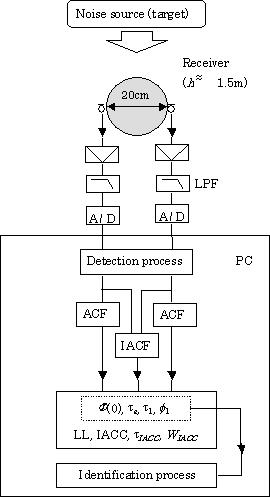Dual-channel electrostatic microphones are used as the receiver, and a sphere between the microphones is used as a simple dummy head. Preliminary investigations comparing a human head, a dummy head, and a styrene foam sphere 20 cm in diameter revealed that the physical factors discussed here are not much affected by the shape of the head. The sampling frequency is usually 44.1 kHz and all the orthogonal factors are extracted from the ACF and IACF in real time. The noise source may then be identified by the use of ACF factors as described in section 4. The IACF factors mainly indicate the spatial information like the directivity or diffuseness in relation to the noise source. For further information for other aspects on the system, refer to our web site .

3. CALCULATION OF ORTHOGONAL FACTORS

3.1. PEAK-DETECTION OF ENVIRONMENTAL NOISE

A number of measurement sessions of the environmental noise to be analyzed are extracted by a peak-detection process. In order to automatically extract environmental noises or target noises from a continuous noise, a monoaural energy Fll(0) or Frr(0), which is energy at the left or the right ear entrance, respectively, is continuously analyzed. The peak-detection procedure is shown in Figure 2, and the conditions determined in this analysis are listed in Table 1.

FIGURE 2. Procedure for extracting target noise for a single session. The concept of running integration interval is also presented. Running ACF and running IACF are conducted for every sessions to extract physical factors.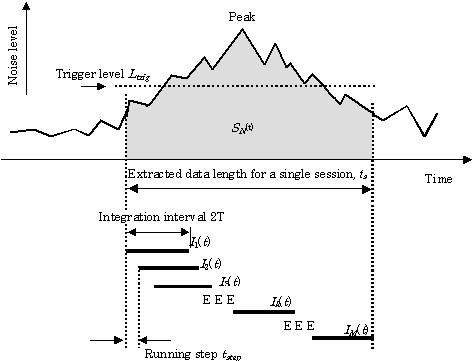TABLE 1
Conditions to be determined in the detection process, the calculation of the running ACF and running IACF, and the extraction of te

 Calculation process Conditions (a) Detection process Trigger level Ltrig (dB) Data length for a single session ts(s) (b) Calculation of running ACF and running IACF Integration interval 2T(s) Running step tstep (ms) (c) Calculation of te Time interval for detecting peaks Dt (ms)

The interval for the calculation of F(0) can be fairly long, say 1 s, when the noise is a continuous one such as aircraft noise or railway noise, but a shorter interval must be used when the noise is brief or intermittent. For the running calculation in equation (1) described below, however, it may be necessary to select an interval longer than the integration interval. Thus, this time interval must be determined according to the kind of the noise source. This enables F(0) to be determined more accurately than it can be determined when using a normal sound level meter with a long time constant. The peaks cannot be detected unless the trigger level Ltrig is properly set in advance. The appropriate Ltrig value also varies according to the kind of target noise, the distances between the target and the receiver, and atmospheric conditions. It must therefore be determined by means of a preliminary measurement. It is easy to determine the value of Ltrig, when the distance between the target and the receiver is short and there is no interfering noise source near the receiver. The noise centered on its maximum F(0) is recorded on the system as a single session. The duration of one session for each target noise, ts, should be selected so as to include F(0) peak after exceeding Ltrig value. For normal environmental noise like aircraft noise and railway noise, the value of ts can be about 10 s. This is different from steady state noise with longer duration or intermittent noise with shorter duration. Note that the present system cannot be used when there are interfering noises. As shown in Figure 2, the set of sessions {S1(t), S2(t), S3(t), ..., SN(t); N: the number of sessions, 0 < t< ts} are stored on the system automatically.
The running ACF and running IACF for each session SN(t) with duration ts are analyzed as shown in the figure. Here we consider only a single session in order to explain the process of "running". Appropriate values for the integration interval 2T and running step tstep are determined before the calculation. As explained in reference , the recommended integration interval seems to be around 30 (
te)min, where (te)min is the minimum value of the running series of values te, and can easily be found by preliminary measurement. This is found by the use of data of different kinds of environmental noises. In most cases, adjoining integration intervals overlap each other. The ACF and the IACF are calculated for every step (n = 1, 2, ..., M) within one session with the range of 2T which shifts in every tstep, as {(0, 2T), (tstep, tstep + 2T), (2tstep, 2tstep + 2T),..., ((M – 1)tstep, (M – 1)tstep + 2T)}. Physical factors are extracted from each step of the ACF and the IACF. Note that 2T must be sufficiently longer than the expected value of te. Also, it should be deeply related to an "auditory time-window" for sensation of each step. A 2T between 0.1 and 0.5 s may be appropriate for environmental noise , but a value near 2.5 s is recommended for music . If 2T is less than this range, the (te)min converges at a certain value. In most cases, the tstep is recommended around 0.1 s. If a more detailed activity of fluctuation is necessary, a shorter tstep should be selected.
As is well known, the ACF and the IACF are analyzed by using the FFT for the binaural signals and then using the inverse FFT. The A-weighting filter and frequency characteristics of microphones must be taken into consideration after the process of FFT.

3.2. ACF FACTORS

The ACFs at the left and right ears are, respectively, represented as Fll (t) and Frr (t). In discrete numbers, they are represented as Fll(i) and Frr(i) (1 < i < Tf ; f : sampling frequency (Hz); i : integer). In the calculation of F(0) for left and right values, Fll(i) and Frr(i) are averaged as follows:. (1)

An accurate value for the SPL is given by

 SPL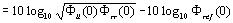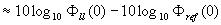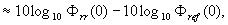(2)

where Fref(0) is the F(0) at the reference sound pressure, 20 mPa. The binaural listening level is the geometric mean of Fll(0) and Frr(0):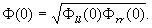(3)

Since this F(0) is the denominator for normalization of the IACF, it can be considered to be calssified as one of the IACF factors: or the right hemispheric spatial factors .
The effective duration,
te, is defined by the delay time at which the envelope of the normalized ACF becomes 0.1 (the 10 percentile delay: see Figure 3).

FIGURE 3.
An example of the calculation of the effective duration, te, from normalized ACF by linear fitting to the initial envelope of the ACF.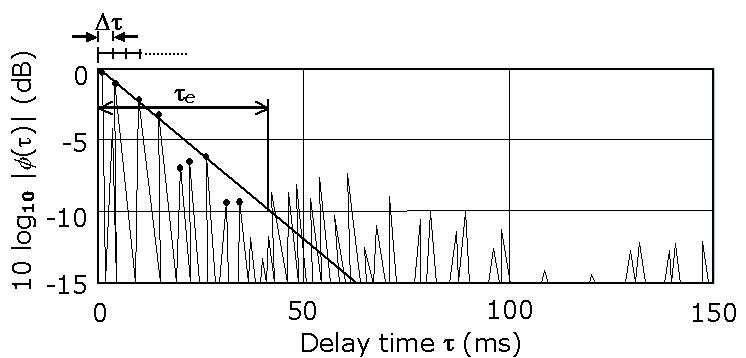The normalized ACF for the left and right ears, fll,rr (t), is obtained as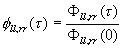(4)

It is easy to obtain te if the vertical axis is transformed into the decibel (logarithmic) scale, because the linear decay for initial ACF is usually observed as shown in the figure. For the linear regression, the least mean square (LMS) method for ACF peaks which are obtained within each constant short time range Dt is used. The Dt is used for the detection of peaks in the ACF and must be carefully determined before calculation. In calculating te, the origin of the ACF ( = 0, at t = 0) is sometimes excluded if it is not in the regression line. As an extreme example, if the target noise consists of a pure tone and a white noise, rapid attenuation at the origin due to the white-noise components is observed, and the subsequent decay is kept flat because of the pure-tone component. In such a case, the envelope function of ACF must be figured out.
As shown in Figure 4, t1 and f1 are, respectively, the delay time and amplitude of the first peak of the normalized ACF. The first maximum must be determined as a main peak avoiding local minor peaks. The factors tn and fn (n > 2) are excluded because they are usually related to t1 and f1.

FIGURE 4. Definitions of t1 and f1 for the normalized ACF.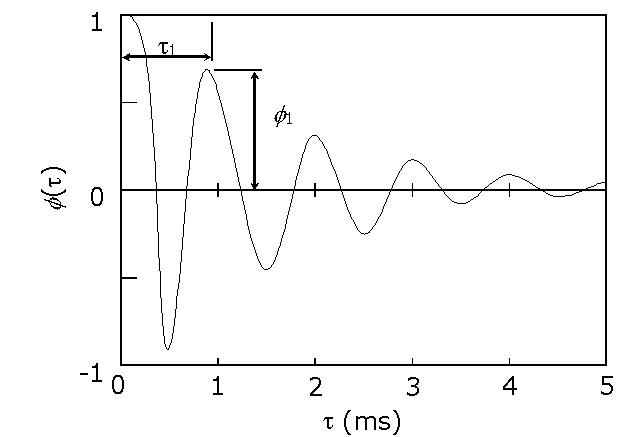3.3. IACF FACTORS

The IACF between sound signals at left and right ears is represented as Flr(t) ( - 1 < t < + 1 (ms)). In the digital form, it is represented as Flr(i) ( - f / 103 < i < f / 103 ; i : integer, where negative values signify the IACF as the left channel is delayed). Thus, it is enough to consider only the range from - 1 to + 1 ms, which is the maximum possible delay between the ears. The IACC is a factor related to the subjective diffuseness. As shown in Figure 5, it is obtained as the maximum amplitude of the normalized IACF flr(i) within the delay range.

FIGURE 5.
Definitions of the IACC, tIACC, and WIACC descriptors from the IACF.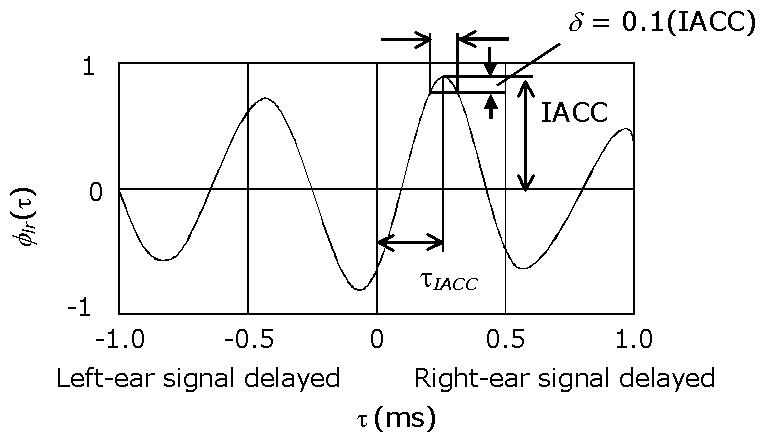Thus,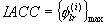. (5)

The normalized IACF is given by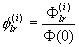. (6)

The value of tIACC is simply obtained at the time delay of the maximum amplitude. For example, if tIACC is greater than zero (positive), the sound source is on the right side of the receiver or is perceived as if it were. As shown in Figure 5, the value of WIACC is given by the width of the peak at the level 0.1 (IACC) below the maximum value. The coefficient 0.1 is approximately used as JND at IACC = 1.0.
The listening level LL is obtained by the manner represented in equation (2) upon replacing SPL with LL.
Thus, physical factors extracted from fine structures of the ACF and IACF are obtained for each integration interval as running values.

4. SOURCE IDENTIFICATION USING THE ACF FACTORS

As shown in Figure 1, noise sources are identified by using four ACF factors in the present stage. Since the F(0) varies according to the distance between the source and receiver, special attention is paid to the conditions for calculation if the distance is unknown. Even if the factor F(0) is not useful, the noise source can be identified by using the other three factors. Remaining IACF factors may be taken into account if the spatial information is changed. One of the guidelines to figure out the minimum te, (te)min, which represents the most active part of the noise signal, is the fact that the piece is most deeply associated with subjective responses . The distances between the values of each factor at (te)min for the unknown target data (indicated by the symbol a in equations (7-10), and values for the template (indicated by the symbol b) are calculated. Here, "target" is used as an environmental noise as an object to be identified by the system. Template values of a set of typical ACF factors for a specific environmental noise are prepared, and these templates for comparison with an unknown noise.
The distance D(x) (x:
F(0), te, t1, and f1) is calculated in the following manner: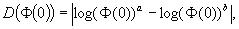(7)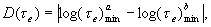(8)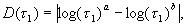(9)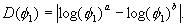(10)

The total distance D of the target can be represented as the sum of the right-hand terms of equations (7)-(10), so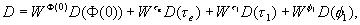(11)

where W(x) (x: F(0), te)min, t1, and f1) signifies the weighting coefficient. The template with the nearest D can be taken as the identified noise source. The method used to compute the weighting coefficients is described in Appendix A.

APPENDIX A: COMPUTATION OF THE WEIGHT COEFFICIENT

Weighting coefficients W(x) (x: F(0), te, t1, and f1) in equation (11) are obtained by using statistical values s1(i) and s2(i). As shown in Figure A1, s1(i) is the arithmetic mean of the standard deviations (SD) for all categories of the ACF factor. Here category means a set of data for the same kind of noise. s2(i) is the SD of the arithmetic means for each category. Values of W(x) are given as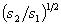after normalization by maximum values among factors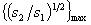. This square root processing is experiential and would be improved by introduction of a better function. The procedure is explained as follows. As a factor with larger SD between noise sources and with smaller SD among a certain source can distinguish the different kinds of noise, the weighting of such factor should be larger than that of the other factors. If the learning function toward the improvement of a template is given, a template is overwritten in order by average values of each ACF factor between the latest session and the previous data in the system.

FIGURE A1. The method to compute the weighting coefficients, W(x) in equation (12).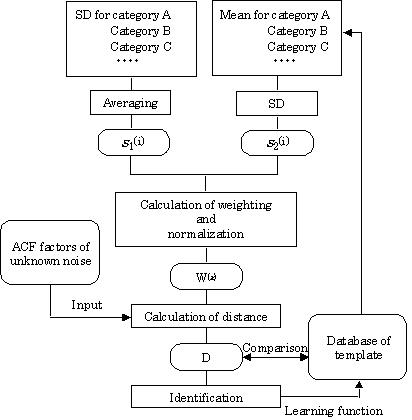5. REMARKS

This paper described the detection of environmental noise, the analysis of ACF and IACF factors, and a process for identifying unknown environmental noises. The computational system described here may be useful for characterizing environmental noises. Such a noise can be identified by using four factors extracted from the ACF: F(0), te, t1, and f1. Though the spatial factors extracted from the IACF (LL, IACC, tIACC, and WIACC) are not used for the identification in this paper, spatial information on the noise source including its degree of diffuseness and its direction from the receiver can be described by these spatial factors. Experimental results which include spatial factors from the IACF are demonstrated in references [11, 12] in this special issue.

ACKNOWLEDGMENTS

The authors would like to thank Mr. Shinichi Aizawa for his invaluable assistance with programming the software. This work was supported by the Research and Development Applying Advanced Computational Science and Technology Program of the Japan Science and Technology Corporation (ACT-JST), 1999.

REFERENCES

 1 Y. ANDO 1998 Architectural Acoustics: Blending Sound Sources, Sound Fields, and Listeners. New York: A1P/Springer-Verlag. 2 I. G. N. MERTHAYASA and Y. ANDO 1996 Japan and Sweden Symposium on Medical Effects of Noise. Variation in the autocorrelation function of narrow band noises; their effect on loudness judgment. 3 S. SATO, H. SAKAI and Y. ANDO in Journal of Sound and Vibration. The loudness of "complex noise" in relation to the factors extracted from the autocorrelation function (to be published). 4 M. INOUE, Y. ANDO and T. TAGUTI in Journal of Sound and Vibration. The frequency range applicable to pitch identification based upon the autocorrelation function model (to be published). 5 K. MOURI, K. AKIYAMA and Y. ANDO in Journal of Sound and Vibration. Preliminary study on recommended time duration of source signals to be analyzed, in relation to its effective duration of autocorrelation function (to be published). 6 Y. ANDO, T. OKANO and Y. TAKEZOE 1989 The Journal of the Acoustical Society of America 86, 644-649. The running autocorrelation function of different music signals relating to preferred temporal parameters of sound fields. 7 Y. ANDO in Journal of Sound and Vibration. A theory of primary sensations measuring environmental noise (to be published). 8 M. SAKURAI, S. AIZAWA and Y. ANDO 1999 The Journal of the Acoustical Society of America 105, 1369. An internet-oriented system for acoustic measurements of sound fields. 9 Web site of Yoshimasa Electronic Inc. (URL: http://www.ymec.co.jp/index.htm). 10 K. MOURI, K. AKIYAMA and Y. ANDO 2000 Journal of Sound and Vibration 232, 139-147. Relationship between subjective preference and the alpha-brain wave in relation to the initial time delay gap with vocal music. 11 H. SAKAI, S. SATO, N. PRODI and R. POMPOLI in Journal of Sound and Vibration. Measurement of regional environmental noise by use of a PC-based system: an application to the noise near the airport 'G. Marconi' in Bologna (to be published). 12 K. FUJII, Y. SOETA and Y. ANDO in Journal of Sound and Vibration. Acoustical properties of aircraft noise measured by temporal and spatial factors (to be published).